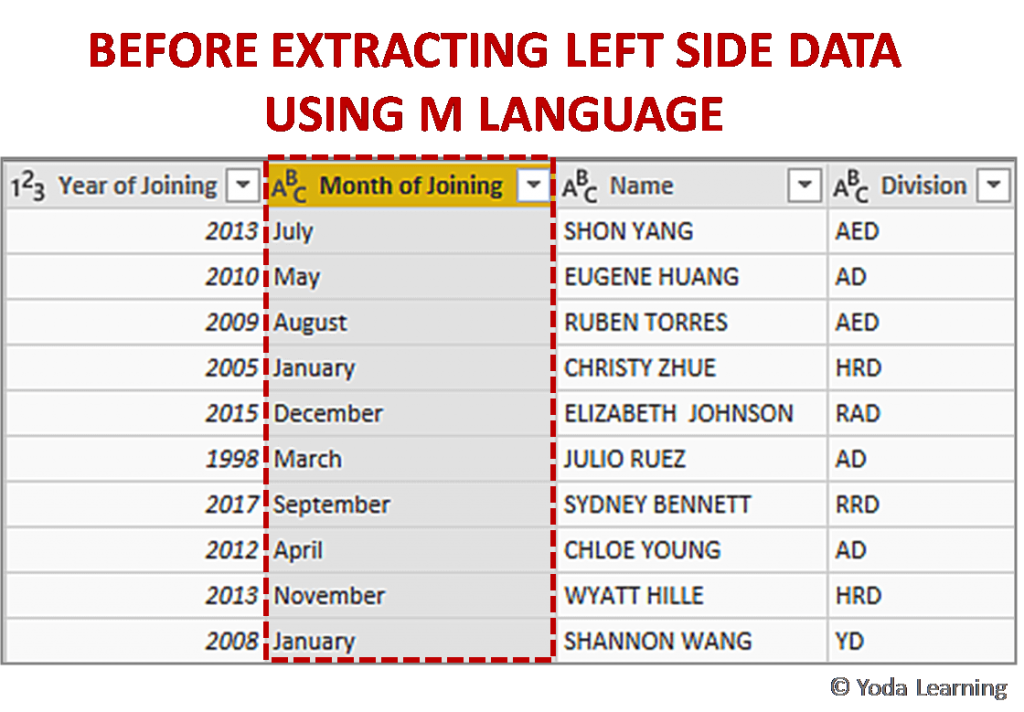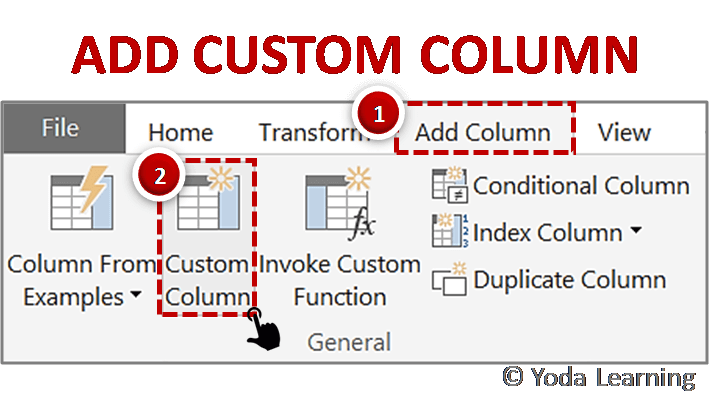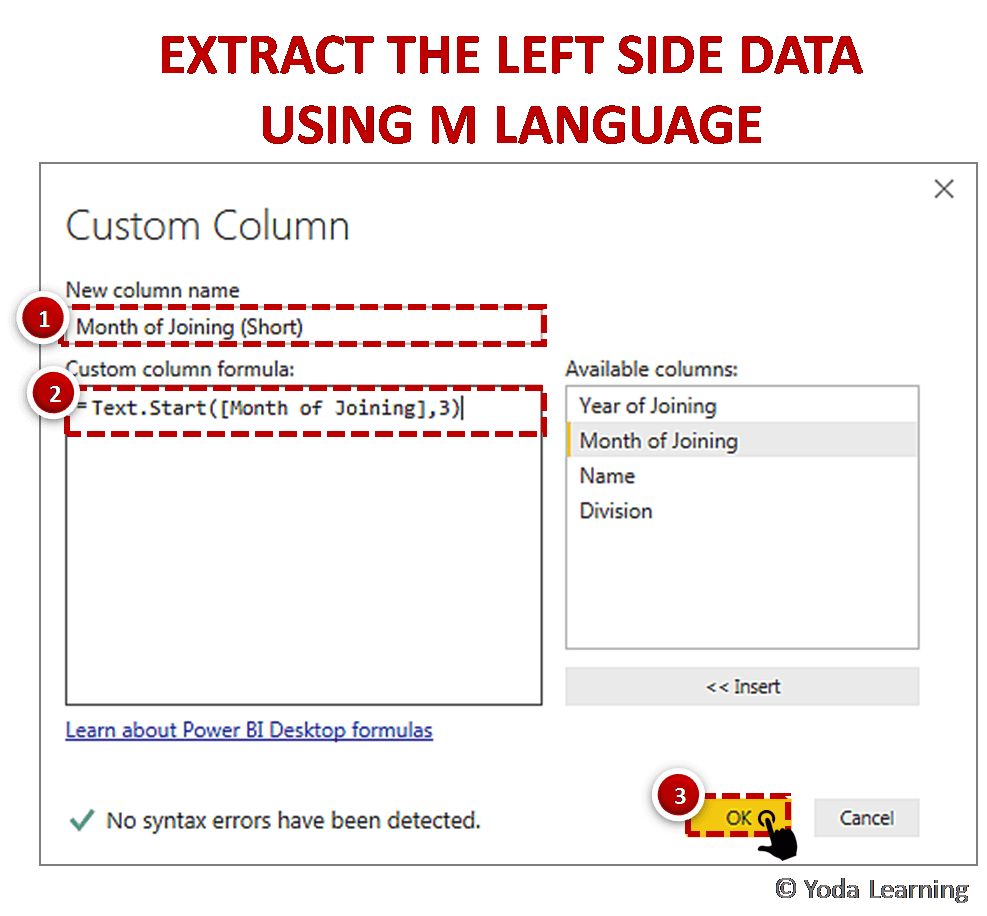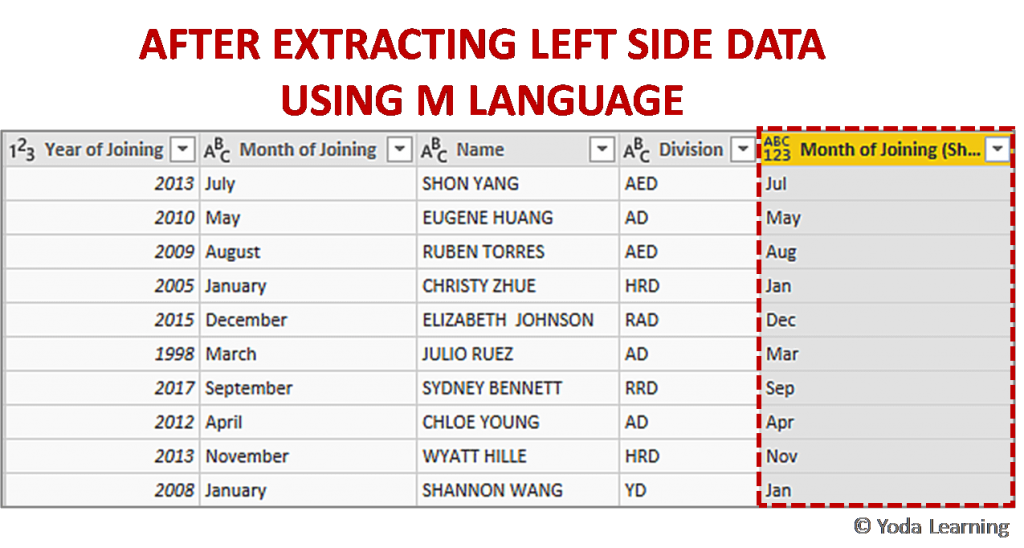# Use Excel’s Right Function With ‘M’ To Extract Left Side Data

If you want to use the Excel LEFT Function in Power Query, then you can’t directly go for it. This is because the Excel LEFT Function is not supported in M.

Data Analytics Tricks in Power BI – Guidebook

You too can analyze data like a Data Scientist. No coding needed. No statistics needed.

Analyze & Visualize data using Power BI. (23 tricks in one book)

## Use Excel’s Right Function With ‘M’ To Extract Left Side Data

But there is an alternate way for using the LEFT Function in M which is explained below.

### Step 1: Select the Column for Extracting Left Side Data

Load the data in Power Query and select the ‘Month of Joining’ column for applying custom Column formula as shown in below picture.### Step 2: Add Custom Column to Extract Left Side Data

To add the Custom Column go to-### Step 3: Extract Left Side Data using M Language

To extract the left side data we have to create a simple M expression.

In the New column name text box, we have to write the new column name as shown in the below picture.

Now, you can write the M query as given below:

• In the Custom column formula, type the function- Text.Start(
• From the Available columns select the Month of Joining column and press Insert.
• Then complete the formula by entering ‘, 3)’

The final formula looks like this:

Text.Start([Month of Joining], 3)

Where,

• Start function is to get the first X characters of the Month of Joining column
• Number 3 is used to specify the first 3 characters (left side characters) to be extracted as shown below.### Step 4: Close & Apply

Close and apply the changes after extracting left side data as shown in the below picture.Data Analytics Tricks in Power BI – Guidebook

You too can analyze data like a Data Scientist. No coding needed. No statistics needed.

Analyze & Visualize data using Power BI. (23 tricks in one book)